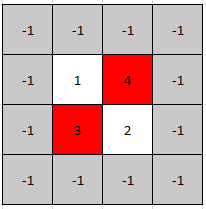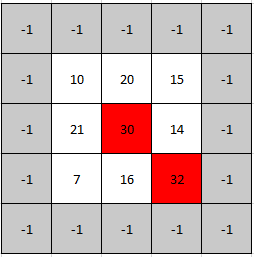# GeetCode Hub

A peak element in a 2D grid is an element that is strictly greater than all of its adjacent neighbors to the left, right, top, and bottom.

Given a 0-indexed `m x n` matrix `mat` where no two adjacent cells are equal, find any peak element `mat[i][j]` and return the length 2 array `[i,j]`.

You may assume that the entire matrix is surrounded by an outer perimeter with the value `-1` in each cell.

You must write an algorithm that runs in `O(m log(n))` or `O(n log(m))` time.

Example 1:```Input: mat = [[1,4],[3,2]]
Output: [0,1]
Explanation: Both 3 and 4 are peak elements so [1,0] and [0,1] are both acceptable answers.
```

Example 2:```Input: mat = [[10,20,15],[21,30,14],[7,16,32]]
Output: [1,1]
Explanation: Both 30 and 32 are peak elements so [1,1] and [2,2] are both acceptable answers.
```

Constraints:

• `m == mat.length`
• `n == mat[i].length`
• `1 <= m, n <= 500`
• `1 <= mat[i][j] <= 105`
• No two adjacent cells are equal.

class Solution { public int[] findPeakGrid(int[][] mat) { } }